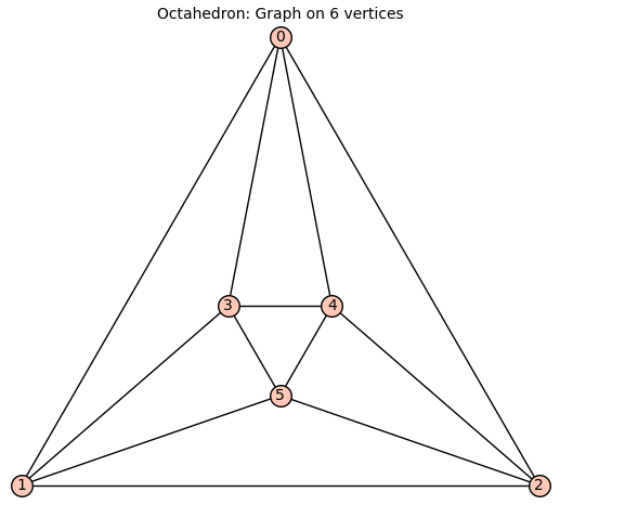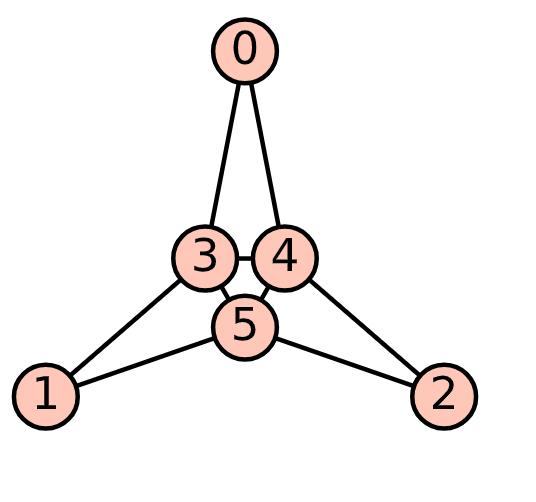# subgraph_search_iterator gives duplicate subgraphs

I use subgraph_search_iterator to obtain all the subgraphs isomorphic to $H$ of a graph $G$. However, in the following example, I found that many obtained subgraphs appear to be duplicates, which surprises me. Have I misunderstood this function?

G=graphs.OctahedralGraph()
H = graphs.CycleGraph(3)
L = list(G.subgraph_search_iterator(H, induced=False,return_graphs=False))
len(L)L
L
L


We will see that the 3-cycle formed by vertices 0, 1, and 3 appears three times. What could be the reason for this? How can we avoid such occurrences?

Edits: In fact, the 3-cycle formed by vertices 0, 1, and 3 appears six times.

Perhaps the $C_3$ are too specific and overshadowing something. If we consider the following example, we will discover that $H$ appears as many times as the number of copies of H without labels multiplied by the order of automorphisms of $H$. (label $H$ ?)

G=graphs.OctahedralGraph()
H=copy(G)
H.delete_edges( [(0,1), (1,2), ( 0,2 )])
print( sum(1 for _ in G.subgraph_search_iterator(H, induced=False,return_graphs=False) ) )
L = { tuple(g.edges(labels=False,sort_vertices=True,sort=True)) for g in G.subgraph_search_iterator(H, induced=False) }
print(len(L))
H.automorphism_group( ).order( )48
8
6

edit retag close merge delete

Sort by » oldest newest most votedIt looks like a bug. Please submit a bugreport at https://github.com/sagemath/sage/issues

Meanwhile, a workaround would be to store subgraphs as sorted tuples of edges in a set, which will eliminate the duplicates:

G=graphs.OctahedralGraph()
H = graphs.CycleGraph(3)
print( sum(1 for _ in G.subgraph_search_iterator(H, induced=False,return_graphs=False) ) )
L = { tuple(g.edges(labels=False,sort_vertices=True,sort=True)) for g in G.subgraph_search_iterator(H, induced=False) }
print(len(L))


This prints 48 and 8, meaning that among the 48 reported subgraphs only 8 are unique.

more

1

Why it was made that way is a mystery to me as well. It appears to be giving back all possible $H$-automorphisms. I would submit a bugreport. See https://github.com/sagemath/sage/issu...

1

Looks like it was meant to return pairs (S,f) where S is a subgraph of G and f is a bijective mapping of H into S. However, it returns only S. The duplicates correspond to different ways to map H into S.

In your example: H is a 3-cycle, it can be mapped to another 3-cycle in 6 different ways. Hence, we have $48 = 8\cdot 6$.

Yes. Indeed. It appears not only three times but six times.